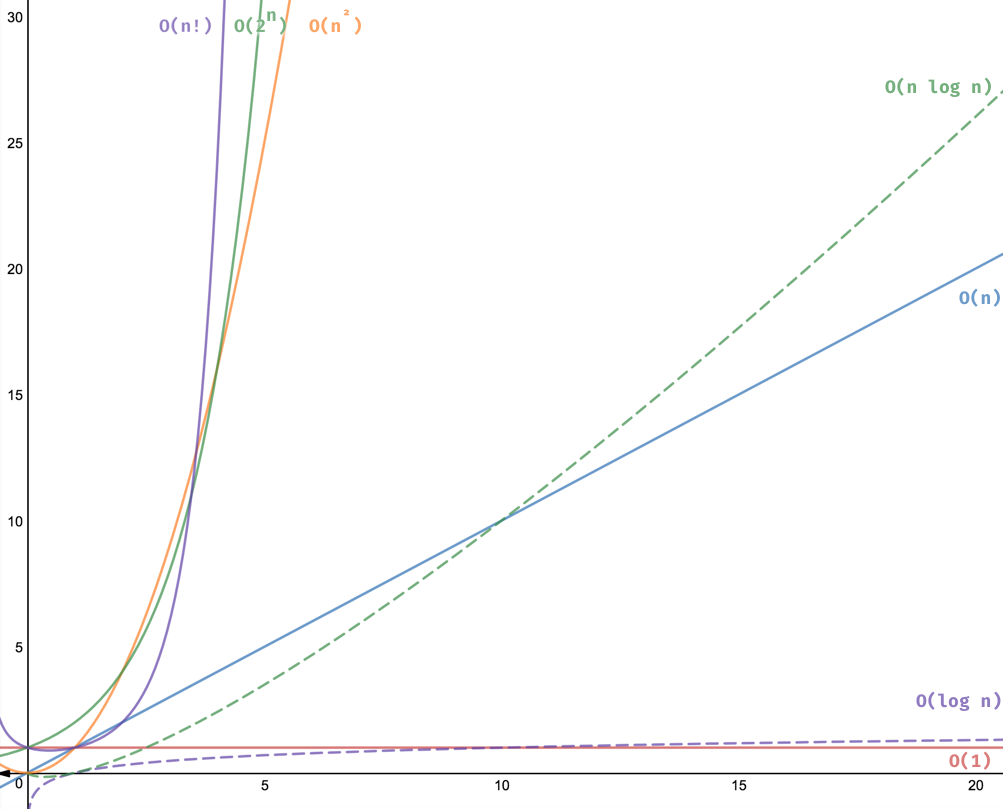# CSC300: Counting, Approximation, Time Complexity, and Big Oh

 Contents [0/9]

 Video [1/9] Counting and exponentiation [2/9] Counting and logarithm [3/9] Fun facts [4/9] Common numbers [5/9] Common numbers [6/9] Changing the base [7/9] Approximation [8/9] Order of growth [9/9]

(Click here for one slide per page)

 Video [1/9]

In two parts

 Open Playlist
 Open Playlist

 Counting and exponentiation [2/9]

We are all used to counting by adding one (arithmetic series):

```0 1 2 3 4 5 6 7 ... N
```

You can also count by multiplying by two (geometric series):

```1 2 4 8 16 32 64 128 ... N
```

There is correspondence between these two forms of counting, called exponentiation.

```      2^0  =    1       2^10 = 1024   ~ thousand    (kilo/kibi)
2^1  =    2       2^20 = 1024^2 ~ million     (mega/mebi)
2^2  =    4       2^30 = 1024^3 ~ billion     (giga/bigi)
2^3  =    8       2^40 = 1024^4 ~ trillion    (tera/tebi)
2^4  =   16       2^50 = 1024^5 ~ quadrillion (peta/pebi)
2^5  =   32       2^60 = 1024^6 ~ quintillion (exa/ exbi)
2^6  =   64
2^7  =  128
2^8  =  256
2^9  =  512
2^10 = 1024
```

 Counting and logarithm [3/9]

We are all used to counting by adding one (arithmetic series):

```0 1 2 3 4 5 6 7 ... N
```

You can also count by multiplying by two (geometric series):

```1 2 4 8 16 32 64 128 ... N
```

There is an inverse operation, called logarithm. People often write `lg` for the base 2 logarithm.

```     lg(1) =    0     lg(thousand)    ~ 10
lg(2) =    1     lg(million)     ~ 20
lg(4) =    2     lg(billion)     ~ 30
lg(8) =    3     lg(trillion)    ~ 40
lg(16) =    4     lg(quadrillion) ~ 50
lg(32) =    5     lg(quintillion) ~ 60
lg(64) =    6
lg(128) =    7
lg(256) =    8
lg(512) =    9
lg(1024) =   10
```

 Fun facts [4/9]

These are equivalent:

```  N = 2^k
lg(N) = k
```

Log and exponentiation are inverses:

```  lg(2^k) = k
2^lg(N) = N
```

Breaking up exponents and logs:

```
2^(k+j) = 2^k * 2^j
lg(N*M) = lg(N) + lg(M)
```

 Common numbers [5/9]

Since

```  2^(k+j) = 2^k * 2^j
```

we have:

```   2^32 = 2^2 * 2^30 ~  4 billion     (actually              4_294_967_296)
2^64 = 2^4 * 2^60 ~ 16 quintillion (actually 18_446_744_073_709_551_616)
```

 Common numbers [6/9]

Since

```  lg(N*M) = lg(N) + lg(M)
```

we have:

```  lg(4 billion)      ~ lg(4)  + lg(billion)     = 2 + 30
lg(16 quintillion) ~ lg(16) + lg(quintillion) = 4 + 60
```

 Changing the base [7/9]

In general, logs can have any base. Generally, we write `log_b`, where `b` is the base.

```  log_b(N) = log_a(N) / log_a(b)
```

For example, where `lg(n) = log_2(n)`:

```  log_1024(N) = lg(N) / 10     --- since lg(1024) = 10
```

Another example, where `log(n) = log_10(n)`:

```  log(N) ~ lg(N) / 3           --- since lg(10) = 3.32192809489
```

This video might be helpful to some:

 Open Playlist

Here's a relaxing intro to logarithms you might like:

 Open Playlist

 Approximation [8/9]

Often details are not important

We write `f(n) ~ g(n)` if `f` and `g` are more or less the same

We say `f(n)` is `O(g(n))` if `f(n) ~ a * g(n)`, for some constant `a`

• Called order of growth
• Called time complexity
• Called Big Oh

 Order of growth [9/9]

Name Function Intuition
Constant O(1) Data independent
Logarithmic O(lg(n)) Iteratively cut in half
Linear O(n) Iterate once for each item
Linearithmic O(n * lg(n)) Nested iteration: once linear, once logarithmic
Quadratic O(n^2) Nested iteration: twice linear
Cubic O(n^3) Nested iteration: three times linear
Exponential O(2^n) Combinations
Factorial O(n!) PermutationsImage from 8 time complexities that every programmer should know by Adrian Mejia

Revised: 2008/03/17 13:01